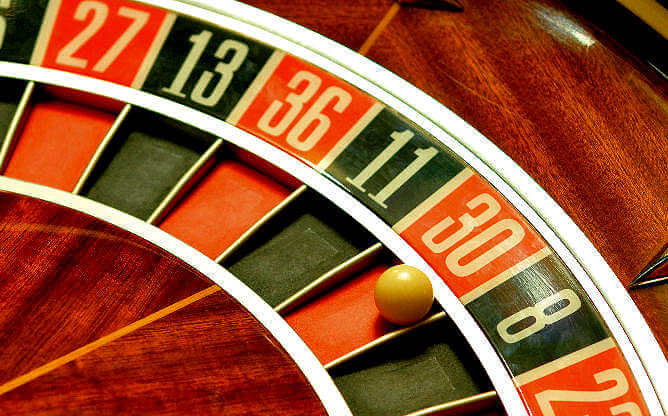It is a very common tactic to bet on five roulette numbers per spin. There is also an automated Five Numbers Bet, that is called Neighbors bet. In the Neighbors bet, you bet a number and its four neighboring numbers: the two numbers on the left to the main number and the two numbers on the right, based on the European wheel layout.

For example the “36 and the Neighbors” bet, includes the numbers: 30, 11, 36, 13, 27. The “17 and the Neighbors” bet includes the numbers: 6, 34, 17, 25, 2. These bets are played with 5 units. One unit for each number.

Let us now examine what one should expect when using a Five Number bet (betting five numbers) continuously and consistently. It doesn’t matter if one bets neighbor numbers or numbers that are far away from each other. It doesn’t matter if one bets the same five numbers every time, or if he changes his selection of five numbers. And it doesn’t matter if one bets in the same table the same day or on various tables and different days. The expectations are the same, as long as one keeps betting five numbers each spin.These 5 neighbor bets win when number 30 comes: 36, 11, 30, 8 and 23 and neighbors,

First we must understand two important concepts: Expectation and Probability

Expectation is much more simple to calculate and it gives a much better understanding of how rare an event is and what to… expect. It tells us the frequency of an event.

Probability is much more complex to calculate and doesn’t offer very useful information on complex systems. It just shows the uncertainty of the event. Uncertainty is inherent to the roulette wheel anyway. That’s why in roulette no event can have 100% probability.

Let us analyze the Five numbers bet on the European wheel (37 numbers).
We keep betting five numbers every spin.
We win if one of our 5 numbers comes and we lose if any of the rest 32 numbers comes.

### Probability of (at least one) win

In one spin: 1-(32/37)= 5/37 = 0,14 = 14%
In two spins: 1-(32/37)x(32/37) = 1-0,75 = 0,25 = 25% (11% increase)
In three spins: 1-(32/37)^3 = 1-0,65 = 0,35 = 35%
In four spins: 1-(32/37)^4 = 1-0,55 = 0,45 = 45%
In five spins: 1-(32/37)^5 = 1-0,48 = 0,52 = 52%
In six spins: 1-(32/37)^6 = 1-0,41 = 0,59 = 59%
In seven spins: 1-(32/37)^7 = 1-0,35 = 0,65 = 65%
In eight spins: 1-(32/37)^8 = 1-0,30 = 0,7 = 70% (5% increase)

We see that the more spins we play the probability of a success increases but in a lower rate as we add more spins. This shows that no matter how many spins we play the probability will increase but it will never reach 100%. THEORETICALLY we can never be 100% sure that our five numbers will hit.

### Expectation of win

In one spin: 5/37 = 14%
In two spins: 2×5/37 = 27%
In three spins: 3×5/37 = 40%
In four spins: 4×5/37 = 54%
In five spins: 5×5/37 = 68%
In six spins: 6×5/37 = 81%
In seven spins: 7×5/37 = 95%
In eight spins: 8×5/37 = 108%

We see that with every spin the expectation increases by 5/37 (or 14%). In the 8th spin the expectation exceeds 100%. This means that in eight spins we “expect” just a bit more than one hit.

There are 37 numbers on the wheel and we bet on 5 of them.
37/5 = 7,4 This means that we should expect our five numbers to hit once every 7,4 spins.
Now it is easy to calculate more expectations.

In how many spins should we expect 12 hits?
Easy: 7,4 x 12 = 88,8 spins.

In how many spins do we expect a repetition of one of our five numbers?
The expectation of a repetition for one number is once every 37 appearances. We play 5 numbers. We would have 37 appearances in 37×7,4= 273,8 spins. In 273,8 spins we expect one of our five numbers to repeat (come two times back to back).

In how many spins do we expect one of our numbers to be followed be another one of our numbers?
Our numbers hit every 7,4 spins. After a hit, the expectation of a repetition of our numbers is 1/7,4. So a repetition is expected after 7,4 appearances of our numbers. 7,4 x 7,4 = 54,7 spins. Every 54,7 we expect 7,4 appearances of our numbers and two of them are expected to be back to back.

It is kind of absurd to talk about spins and using decimal numbers. But the whole point of calculating expectation is NOT to find out when a specific even will appear, but to get an idea of its frequency. Event frequency is very important in planing your attack. Expected frequency is a more useful kind of information than probability.

The above short analysis is just a simple example what a player must know before applying a specific attack. This knowledge helps the player to understand what should happen, to evaluate the deviations of the real world data from the theoretical expectation and to be flexible to respond accordingly.